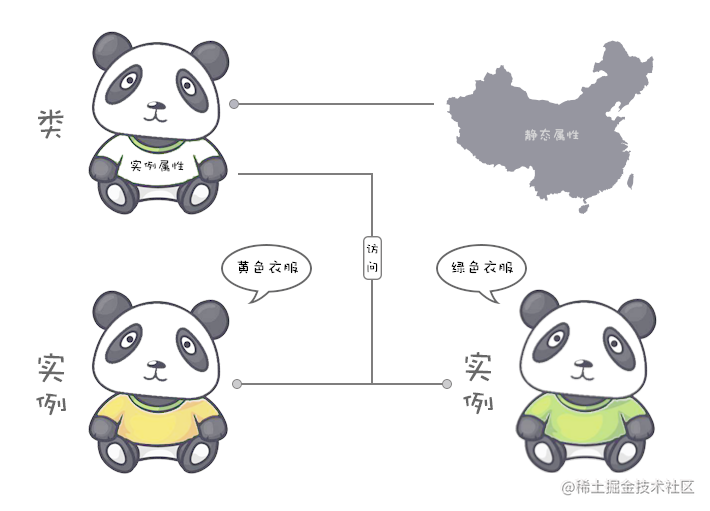# TypeScript 面向对象中的属性和方法

## 属性和方法

``````class Cat {
// 属性
name;

// 方法
sayHi() {
return `Meow, my name is \${this.name}`;
}
}

let tom = new Cat();
tom.name = 'Tom';

console.log(tom.sayHi());
// Meow, my name is Tom

``````class Cat {
// 属性
name: string;

// 方法
sayHi(): string {
return `Meow, my name is \${this.name}`;
}
}

let tom = new Cat();
tom.name = 'Tom';

console.log(tom.sayHi());
// Meow, my name is Tom

### 习题：关于类的属性和方法，下面用法正确的是？

``````// A
class Animal {
age = 10;
getAge() {
return Animal.age;
}
}
new Animal().getAge();

// B
class Animal {
age = 10;
getAge() {
return this.age;
}
}
new Animal().getAge();

// C
class Animal {
age = 10;
getAge() {
return this.age;
}
}
new Animal().age;

// D
class Animal {
age = 10;
getAge() {
return this.age;
}
}
Animal.age;

``````new Animal()

``````new Animal().getAge()

``````Animal.age• A - `age` 是类的实例属性，选项中 `Animal.age` 是静态方法的调用方式。故错误。
• B - 选项中使用 `this.age` 是正确的。 `this` 指向实例对象。
• C - 选项中 `new Animal().age` 调用是正确的。因为 `age` 是类的实例属性。
• D - 基于 `C`选项可以看出，此选项是错误的。

## 资料：值为箭头函数的属性

``````class Person {
name: string
age: number

constructor(name: string, age: number) {
this.name = name;
this.age = age;
}

logName = function(name: string) {
console.log('my name is ' + name)
}

getName = function() {
this.logName(this.name)
}
}

const p1: Person = new Person('Richard', 12);
const { getName } = p1; // 通过对象的解构赋值，提取出 getName

p1.getName(); // my name is Richard
getName(); // TypeError: this.logName is not a function

``````class Person {
name: string
age: number

constructor(name: string, age: number) {
this.name = name;
this.age = age;
}

logName = function(name: string) {
console.log('my name is ' + name)
} // 利用 bind 方法为 logName 绑定作用域

getName = function() {
this.logName(this.name)
}.bind(this)
}

const p1: Person = new Person('Richard', 12);
const { getName } = p1; // 通过对象的解构赋值，提取出 getName

p1.getName(); // my name is Richard
getName(); // my name is Richard

``````class Person {
name: string
age: number

constructor(name: string, age: number) {
this.name = name;
this.age = age;
}

logName=function (name: string)  {
console.log('my name is ' + name)
} // 利用箭头函数为 logName 绑定作用域

getName = () =>{
this.logName(this.name)
}
}

const p1: Person = new Person('Richard', 12);
const { getName } = p1 // 通过对象的解构赋值，提取出 getName

p1.getName(); // my name is Richard
getName(); // my name is Richard

## 资料：存取器

``````class User {
constructor(name: string) {
this.name = name;
}
get name() {
return 'Evan';
}
set name(value) {
console.log('setter: ' + value);
}
}

let a = new User('Peter'); // setter: Peter
a.name = 'Richard'; // setter: Richard
console.log(a.name); // Evan

## 类的构造函数

``````class Cat {
// 属性
// name: string;
// 这里可以定义好传入参数的类型
constructor(name: string) {
this.name = name;
}

// 方法
sayHi(): string {
return `Meow, my name is \${this.name}`;
}
}

let tom = new Cat('Tom');
// tom.name = 'Tom';

console.log(tom.sayHi());
// Meow, my name is Tom

## 资料：参数属性

``````class User {
name: string;
age: number;
constructor() {
//...
}
}

``````class User1 {
constructor(public name: string = 'Richard', private age: number = 18) {
//...
}
}

### 习题：关于类的构造函数，下面描述正确的是？

A. 主要用于初始化类的成员变量属性。

B. 类的对象创建时自动调用执行。

C. 没有返回值。